# Python tkinter image icons

Basics of Python Tkinter

## Changing the default icon

There is a default icon comes with the window.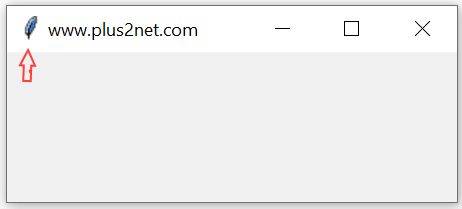``````import tkinter as tk
my_w=tk.Tk()
my_w.geometry('300x100')
my_w.title('www.plus2net.com')
my_w.mainloop()``````

Tkinter displaying icon or JPG PNG image in windows by using Label or button using PILLOW library

Escape the path by using two backslashes if you have any char with can be used with backslash. Here `\f` can be understood as form feed, so we used two backslashes.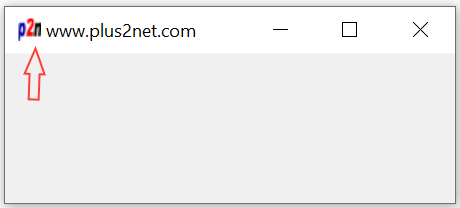``````import tkinter as tk
my_w=tk.Tk()
my_w.geometry('300x100')
my_w.title('www.plus2net.com')
my_w.iconbitmap('D:\\images\\favicon.ico')
my_w.mainloop()``````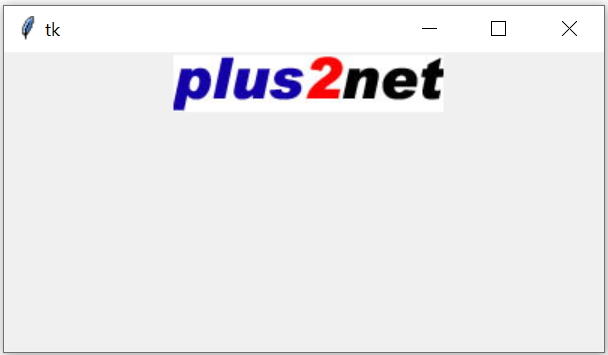``````import tkinter  as tk
my_w = tk.Tk()
my_w.geometry("400x200")

l1 = tk.Label(my_w,  width=15 )
l1.grid(row=1,column=1)

my_img = tk.PhotoImage(file = "D:\\top2.png")
l2 = tk.Label(my_w,  image=my_img )
l2.grid(row=1,column=2)

my_w.mainloop()``````
You may change you path based on the location of the image file in your system.

## Using a Button to add image.

We can place image over one Button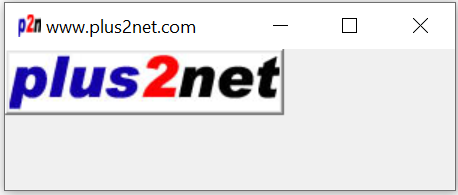``````import tkinter as tk
my_w=tk.Tk()

my_w.geometry('300x100')
my_w.title('www.plus2net.com')
my_w.iconbitmap('D:\\images\\favicon.ico')
my_img = tk.PhotoImage(file = "D:/images/top2.png")

b1=tk.Button(my_w,image=my_img)
b1.grid(row=1,column=1)
my_w.mainloop()``````
Read more on how to disply MySQL Blob binary data (image) using button

## PIL ( Python Image Library)

For .jpg images we have to use PIL library.
``from PIL import ImageTk, Image``
If PIL ( Python Image Library ) is not installed then use PIP to install it. You can check the status by using this command and check all installed libraries.
``%pip freeze``
Here is the code to display Jpg image over a button.
``````import tkinter as tk
my_w=tk.Tk()
from PIL import Image,ImageTk
my_w.geometry('300x100')
my_w.title('www.plus2net.com')
my_img = ImageTk.PhotoImage(Image.open("D:/images/top2.jpg"))
b1=tk.Button(my_w,image=my_img)
b1.grid(row=1,column=1)
my_w.mainloop()``````

## Background Image of the window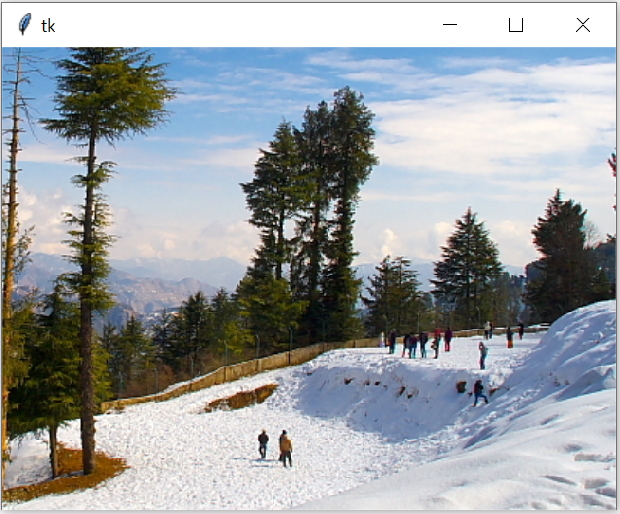Change the path of the image used at my_img2.
To use JPG image we have to include PIL library.
``````import tkinter  as tk
from tkinter import ttk
from PIL import Image,ImageTk
my_w = tk.Tk()
my_w.geometry("840x570")
#my_img = tk.PhotoImage(file = "D:\\top2.png")
my_img2 = ImageTk.PhotoImage(Image.open("D:\\my_data\\background1.jpg"))

bg = tk.Label(my_w, image=my_img2)
bg.place(x=0, y=0, relwidth=1, relheight=1)

my_w.mainloop()``````
For PNG image no need of PIL library.
``my_img = tk.PhotoImage(file = "D:\\top2.png") ``

## Subscribe

* indicates required
Subscribe to plus2netplus2net.com